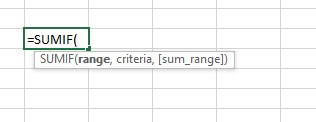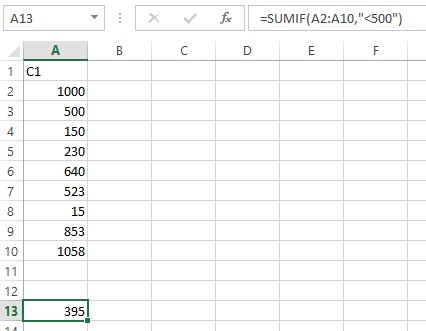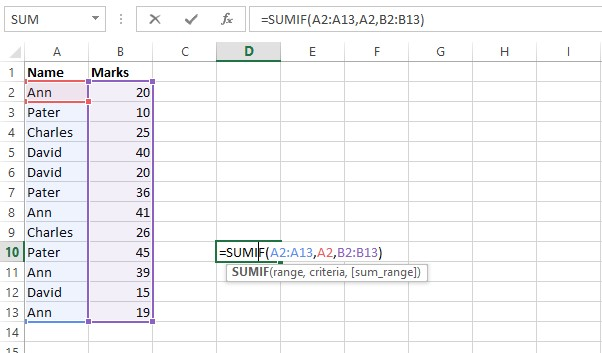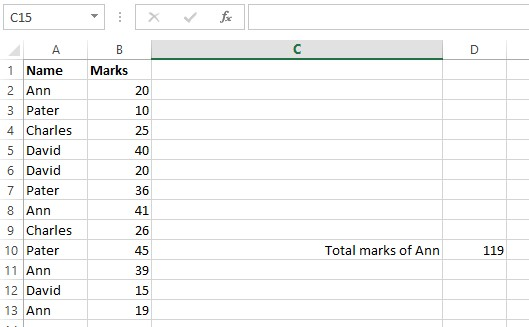# How to Use SumifThe SUMIF function is used to conditionally sum values based on a single criteria.

The Syntax of this SUMIF function can be explained as follows:`=SUMIF(range, criteria [sum_range])`

Here, ‘`range``’` refers to the cells that you want to be analyzed by the ‘`criteria`.

`criteria` refers to the condition that specifies which items are to be added. ‘`criteria` can be a number, expression or a text string.

`sum_range`’ is an optional argument, it specifies the cells to be added. If ‘`sum_range`’ argument is omitted then SUMIF treats ‘`range`’ as ‘`sum_range`’.

### Simple worked example

For instance, if you want to sum the numbers that are larger less than 500. You can use this function with the following simple formula: =SUMIF(A2:A10,"<500")This can be used in different ways to suit the requirements in day to day and professional uses.

### Example 2Let’s think that we want to sum marks of Ann from the marks table shown above.

Range:

The first parameter in the SUMIF function is the range of cells that you want to apply the criteria against.

In this example, the first parameter or the range is A2:A13. This is the range of cells or the names of students that will be evaluated to determine if they meet the criteria.

Criteria:

The second parameter or criteria in the SUMIF function is the name of student we want (i.e. Ann) that will be applied against the range, A2:A13.

In this example, the criteria is A2, which contains the name Ann. This is a reference to the cell A2 which contains the text ‘Ann’. The SUMIF function will test each value in A2:A13 to see if it match with Ann.

Sum_range:

The third parameter in the SUMIF function is the range of numbers that will be summed together.

In the above shown example, the third parameter is B2:B13. For every value in A2:A13 that matches A2, the corresponding value in B2:B13 will be summed.